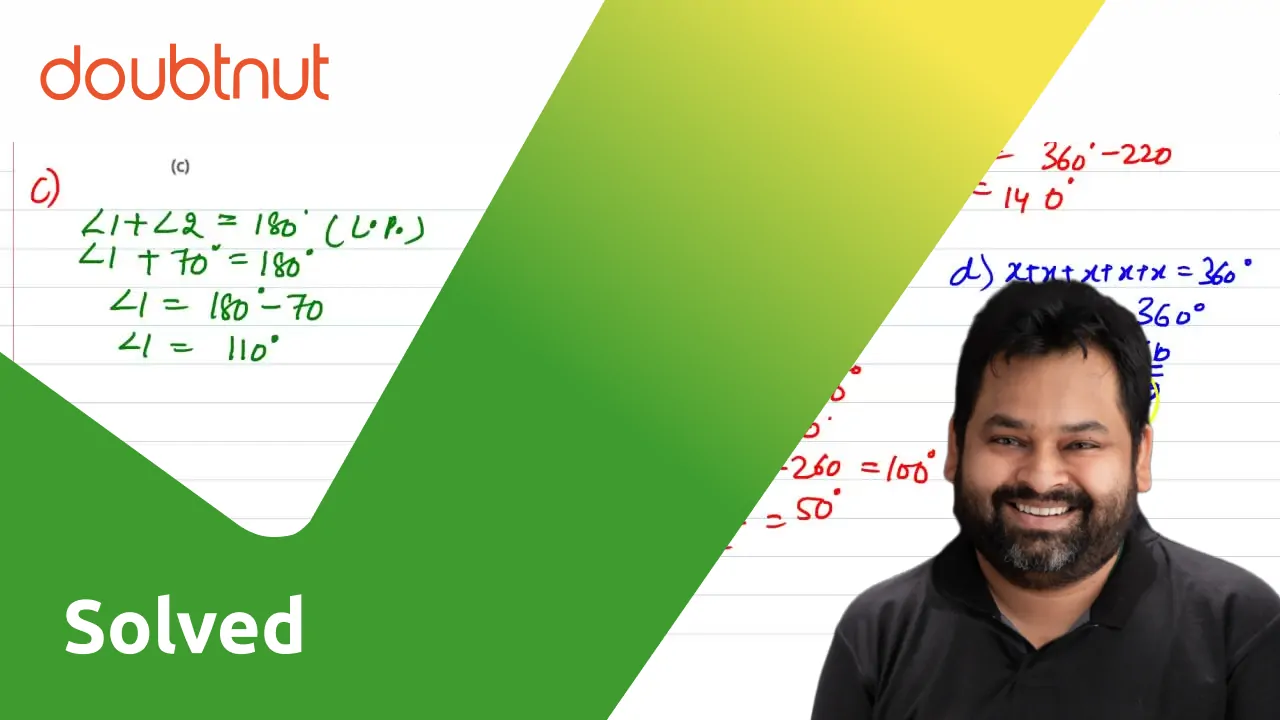Doubtnut App - Get 50 Lakh+ video solutions better than GoogleInstall Now

# Find the angle measure x in the following figures.

Text Solution
Verified by Experts

## (a) The given figure has 4 sides and hence it is a quadrilateral Find the angle measure x Using the angle sum property of a quadrilateral,50∘+130∘+120∘+x=360∘300∘+x=360∘ x=360∘−300∘ x=60∘ (b) Usingtheanglesumproperty of a quadrilateral.Find the angle measurexinthe following figures:The line is perpendicular to the base line hence, the angle formed is 90°.90∘+60∘+70∘+x=360∘220∘+x=360∘220∘+x=360∘x=360∘−220∘x=140∘ (c) The given figure is a pentagon (number of sides =5)Find the angle measure x in the following figures:Angle sum of a polygon = (n−2)×180∘ =(5−2)×180∘=3×180∘=540∘Sum of the interior angle of pentagon is 540^@ .Angles at the bottom are linear pairs. Thus, First base interior angle,= 180^@ - 70^@ (angle of straight line is 180° )=110∘Also, Second base interior angle,=180∘−60∘ =120∘Angle sum property of a polygon = (n - 2) × 180^@ ⇒30∘+x+110∘+120°+x=540∘⇒2x+260∘=540∘⇒2x=540∘−260∘⇒2x=280∘⇒x=280∘2Therefore, x = 140∘(d) The given figure is pentagon (number of sides =5)Find the angle measure x in the following figures:Sum of the interior angle of pentagon is 540^@ Angle sum of a polygon=(n−2)×180∘=(5−2)×180∘=3×180∘=540∘Let each angle of the polygon be x since it is a regular pentagonAngle sum of a polygon =x+x+x+x+x=540∘5x=540∘x=540∘5x=108∘The givenpentagonisaregular polygoni.e.itisboth‘equilateral’ and ‘equiangular’. Thus, the measure of each interior angle of the pentagon is equal.Hence each interior angle is 108∘